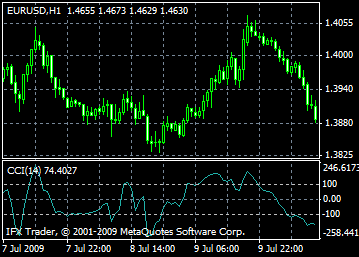# CCI indicator formula and settings: description, adjustment and application

CCI indicator measures deviation of a current price of an instrument from its average price. High values of the CCI indicator suggest that the current price is unusually high comparing to the average price, while low values signal that the current price is too low. Though being named the cci index, this indicator is applicable to any financial instrument.

The CCI indicator can be applied in two ways:

1. For defining deviations

Deviation occurs when a price hits a new peak but the CCI indicator fails to rise above the previous highs. Usually, this classic deviation is followed by a price correction.

2. For defining overbought or oversold zones

Commodity Channel Index usually fluctuates in the range of ±100. Values above +100 indicate the overbought conditions and a possibility of a corrective decline while values below -100 show oversold conditions and a possibility or a corrective rise.Calculation

1. Find the average price. For doing that it is necessary to add the highest and the lowest closing price of every bar and divide the sum by 3:

TP = (HIGH + LOW + CLOSE)/3

2. Calculate the n-period simple moving average of the average prices:

SMA(TP, N) = SUM[TP, N]/N

3. Subtract the obtained SMA(TP,N) from the average prices TP of every preceding n period:

D = TP – SMA(TP, N)

4. Calculate the n-period simple moving average of the absolute values D:

SMA(D, N) = SUM[D, N]/N

5. Multiply the obtained SMA (D, N) by 0,015:

M = SMA(D, N) * 0,015

6. Divide M by D:

CCI = M/D

Where:

HIGH – the maximum price in a bar

LOW – the minimum price in a bar

CLOSE – the closing price

SMA – the simple moving average

SUM – the sum

N – a number of periods used for calculation.

Can't speak right now?